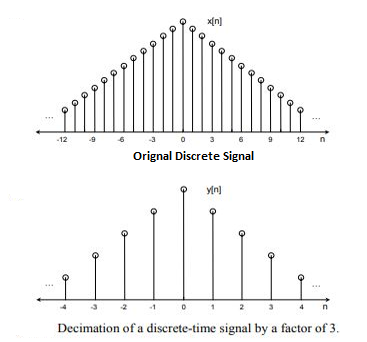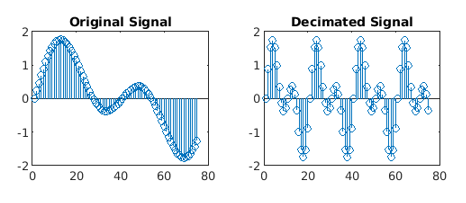# Down-sampling in MATLAB

The two basic operations in a multi-rate system are decreasing/down-sampling (decimation) and increasing (interpolation) the sampling rate of a signal.

In down-sampling we start with a constant time signal x(t) and convert it into a succession of tests x[n], in decimation we start with a discrete-time signal x[n] and convert it into another discrete-time signal y[n], which comprises of sub-tests of x[n].We will be using the decimate() and stem() function.
The decimate() function is used to decrease a sample rate by an integer factor.

Syntax: a = decimate(x, r)
Parameters:

• x: input signal,
• r: decimation factor

Return Value: Decimated Signal

The stem() function is used to plot a discrete sequence data.

Syntax: stem(y)
Parameter:

• y: data sequence

Return Value: Plot of data sequence in discrete time

MATLAB code for down-sampling:

 `% Time vector ` `t = 0 : .00025 : 1; ` ` `  `% Original signal ` `x = sin(2 * pi * 50 * t) + sin(2 * pi * 100 * t); ` ` `  `% Reduces the sample rate of original signal by factor of 4 ` `y = decimate(x, 4);  ` ` `  `figure() ` `subplot(2, 2, 1); ` ` `  `% Plot few samples of the Original signal ` `stem(x(1:75))  ` `title(``'Original Signal'``); ` ` `  `subplot(2, 2, 2); ` ` `  `% Plots few samples of the Decimated signal ` `stem(y(1:75));  ` `title(``'Decimated Signal'``); `

OutputAttention reader! Don’t stop learning now. Get hold of all the important CS Theory concepts for SDE interviews with the CS Theory Course at a student-friendly price and become industry ready.

My Personal Notes arrow_drop_upCheck out this Author's contributed articles.

If you like GeeksforGeeks and would like to contribute, you can also write an article using contribute.geeksforgeeks.org or mail your article to contribute@geeksforgeeks.org. See your article appearing on the GeeksforGeeks main page and help other Geeks.

Please Improve this article if you find anything incorrect by clicking on the "Improve Article" button below.

Article Tags :

2

Please write to us at contribute@geeksforgeeks.org to report any issue with the above content.